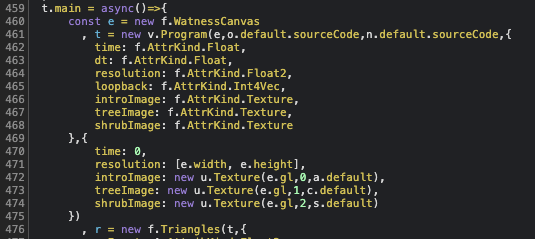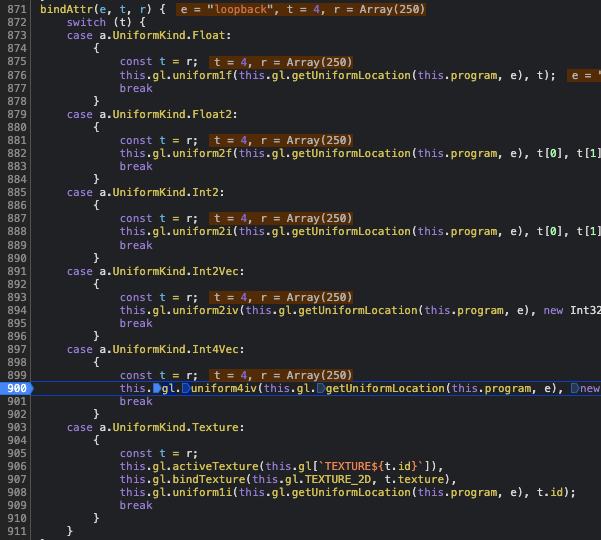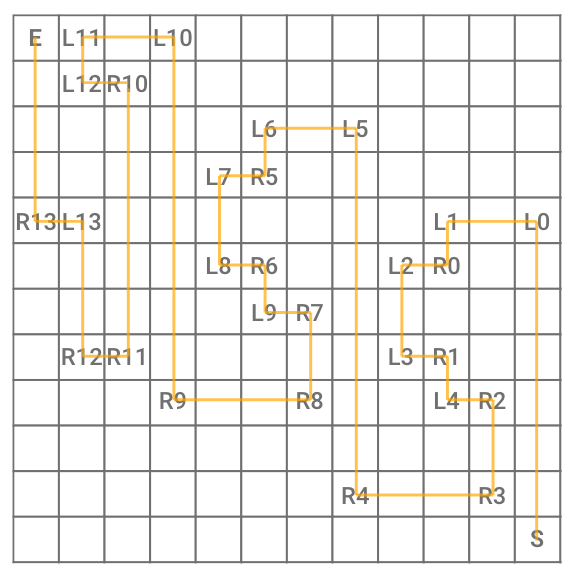# The Watness III [RE, Web]

Reverse engineer a WebGL game by reversing its fragment shader program. First RE and Web challenge from Plaid CTF 2021.

# Challenge Description

“Just when I thought I was out, they pulled me back in again.” From acclaimed writer Jimmy Glow comes the third entry in the Watness trilogy. Our hero is once again pulled into a world of puzzles and problems, with only their wit, persistence, and sheer force of will. Critics rave, “Why have you done this?”

# Exploration

Disclaimer: This writeup will be quite long as I want to go a bit into the details of WebGL or even some working principles of OpenGL. Hopefully it will help you understand how I came up with my solution.

Also, I will be using “WebGL” and “OpenGL” quite interchangeably, since WebGL is just an API to apply OpenGL in the browser.

We are presented with a first-person game and we can draw line paths on a grid, that’s it. So the first thing to do is to open the developer’s console and look at the network and source tabs.

## Finding the WebGL program

The only thing there is `main.js`. After beautifying and studying it, I realized that it’s a WebGL program, with no signs of game logic and/or winning condition. So I figured that I should see how WebGL even works before I can dig deeper.

Essentially, what OpenGL does is it takes each pixel value and passes through a couple of steps, called shaders. You can read on an overview of the rendering pipeline here.

Here, I see `VERTEX_SHADER` and `FRAGMENT_SHADER`, since the JavaScript code doesn’t contain any winning condition check, I thought that it could only be in these two programs.

To find the source code, I first wanted to find where this WebGL program is created, so I looked at `main.js` more and found a main function that acts as the entry.We can put a breakpoint here and trace the two sources

`VERTEX_SHADER` had nothing interesting. Image below shows the export of `FRAGMENT_SHADER`:

The source code looks like `C`, if we consult the OpenGL specifications we can see that it is a thing called OpenGL Shading Language, or GLSL, which is mostly similar to `C`. It has quite a number of built-in types and functions, you can read on it here or get a quick overview.

## Understanding the logic

In this main function, I also found a loop:

It seems complicated, but the important things are lines 533 and 534 in the if-statement. It takes the pixel values and checks whether the x-value of the 150th-indexed pixel is 0 or not (`p` is a point represented by an array of four values which means RGBA or XYZW in GLSL).

Our goal should be getting `p != 0` and running the `alert`. So the flag should be the result of decoding the array related to pixels of index 130 to 147.

We can play around and see how the grid changes this pixel value. Breaking right after `e.readPixels(0, 0, 200)`, and doing a `console.log` of the whole array:

I realized that these corresponded to the line I drew (refer to the image above), in the format of `[x, y, 1, 0]`. Notice in every loop, `p.concat(o)` is set into the `loopback` field of the program, if we trace that line, we can see that this set action triggers a binder that updates the program.I put a breakpoint in the case of Int4Vec because the pixel values are 4-vectors

This breakpoint is important, since it allows us to directly change `loopback` and test things out, remember this for references later on in the writeup. This updated `loopback` will be passed through the shaders to update the pixel values.

To make it easier to read, we can preprocess this code and replace the `#define` directives with the intended expression by running the C preprocessor:

``````cpp source.c clean_source.c
``````

Then, throw it to a beautifier to get better indentations and line breaks. Some important things to note when reading this code:

1. The input is given by `gl_FragCoord`, which is the coordinate of the pixel that this shader is updating, and supplemented by `loopback` and other fields.
2. The output is passed via `gl_FragColor`, which should correspond to the color of the current pixel.
3. The shader starts in `main()`, unsuprisingly…

After inspecting `Cl`, `Ce`, `CN`, I found that `CP` is just the level that we are on. We can verify this by changing `loopback` to 0, 1, 2 respectively: At the breakpoint mentioned earlier, do `t = ...` to update.

Remember our objective? We need `loopback` to not be 0. Looking in `main` we see this near the end:

I found out that `gl_FragCoord.x > 150. && gl_FragCoord.x <= 150. + 1.` just makes sure that the current pixel is indexed 150.

# Solution

So we have our objective here: Make `CP == 3`, but it isn’t as easy as changing the value at that breakpoint, since the final flag is dependent on pixels index 130 to 147.

``````void main() {
...
if (Cs.AB && AD(viewport_center, Cv.v) < 2.) {
CP = CP + 1;
}
...
}
``````

This block suggests that we advance a level every time `Cs.AB == true` (not sure what the other term does, so I ignored it). At level 2, we will have `CP = 2 + 1 = 3` if we solve it!!!

``````struct z {
bool AA;
bool AB;
}; // This essentially is a boolean pair
...
void main() {
...
// We need these to return {XX, true}
z Cs;
if (CP == 0) Cs = Cl(Bo);
if (CP == 1) Cs = Ce(Bo);
if (CP == 2) Cs = CN(Bo);
...
}
``````

## Level 0

``````z Cl(vec2 Bo) {
...
bool Bp = Bj(8);
...
return z(AA, Bp);
}
``````

The value that we want to control is affected by `Bj(8)` only.

``````// Bj(8) => AU = 8
bool Bj(int AU) {
for (int AO = 0; AO < 100 - 1; AO++) {
ivec3 Aj = loopback[int(int(0.) + AO)].xyz;     // Current point
ivec3 Ag = loopback[int(int(0.) + AO + 1)].xyz; // Next point
if (Aj == ivec3(255, 255, 1)) {
return false;
}
if (Aj == ivec3(AU - 1, AU - 1, 1)) {
// We want to reach here to return true
// Aj == [7, 7, 1] which means we should pass through (7,7) in the grid
return true;
}
// Mean of x values of current and next pixel, then dividing by AU = 8
float Bk = (float(Aj.x) + float(Ag.x)) / (2. * float(AU));
// Mean of y values of current and next pixel, then dividing by AU = 8
float Bl = (float(Aj.y) + float(Ag.y)) / (2. * float(AU));
vec4 Bm = texture2D(introImage, vec2(Bk, Bl));
if (Bm.a >= 1.) {
return false;
}
}
return false;
}
``````

I’ve added comments to indicate what each line represents. The thing to take care of is to avoid the case of `Bm.a >= 1.` at all costs, at least before we reach (7, 7).

But what does `texture2D(introImage, ...)` do? According to the description of the function, it takes the pixel of `introImage` at the specified coordinates and returns it.

But from the comments I’ve put, the x and y values are divided by 8, this is because OpenGL wants to normalize the coordinates such that the image dimension doesn’t affect the coordinates that needs to be passed in (read about it here).

And due to normalization, `Bm.a >= 1` means that the alpha value of that pixel is `>= 255`, which essentially means `== 255` since it cannot exceed 255.

Looking around the JS code, we find the image that is represented by `introImage`. I downloaded it and wrote some python code to process it:

``````from PIL import Image

img = Image.open('introImage.png')
result = Image.open('introImage.png')
for x in range(img.width):
for y in range(img.height):
# Pixels are in RGBA format
# Since PIL has (0, 0) at the top-left corner but our grid starts at bottom-left
# we have to invert the y-value of the pixel for the output
if img.getpixel((x, y)) != 255:
result.putpixel((x, y.height-y-1), (0,0,0,255))
else:
result.putpixel((x, y.height-y-1), (255,255,255,255))

result.save('path.png')
``````

The black dots represent the “mid-point” that we can pass through, which means our path should look something like:

And…?

It works! Next level~

## Level 1

Jumping straight to the function for this level:

``````z Ce(vec2 Bo) {
...
bool Bp = Bi(8);
...
return z(AA, Bp);
}
``````

So it’s basically the same thing as last level. We need `Bi(8)` to return `true`.

``````bool Bi(int AU) {
for (int AO = 0; AO < 100 - 1; AO++) {
ivec3 Aj = loopback[int(int(0.) + AO)].xyz; // Current point
if (Aj == ivec3(255, 255, 1)) {
return false;
}
if (Aj == ivec3(AU - 1, AU - 1, 1)) {
// Again, we need to reach (7, 7) in the grid
return true;
}
// AP(y*8 + x, 2) where y*8 + x is sort of indexing the grid points
/*
56 57 58 59 ... 63
.  .  .  . ...  .
8  9 10 11 ... 15
0  1  2  3 ...  7
*/
int R = AP(float(Aj.y * AU + Aj.x), 2.);
// We want the result to be 1 at all times before we return
if (R != 1) {
return false;
}
}
return true;
}
``````

Here’s `AP`, and `AK` which is called in `AP`:

Notice that `AK` is just binary exponentiation, essentially `AK(a,b,m) == pow(a,b,m)` in python. So we can write some code to find which points can be travelled to.

``````# Inverting i because (0, 0) is bottom-left
for i in range(7, -1, -1):
for j in range(8):
AR = pow(1021, 8*i + j + 12, 4093)
AP = AR*2//4093
if AP == 1:
print('O', end='') # Can travel
else:
print('#', end='') # Can't travel
print()
``````

Output:

``````OO##OOOO
O####O##
##OOOOO#
O#####OO
OOO#OOO#
O#OOO###
O##O##O#
OO#OOO#O
``````

## Level 2

``````z CN(vec2 Bo) {
...
bool Bp = BZ(12);
...
return z(AA, Bp);
}
``````

Again, same thing:

``````bool BZ(int AU) {
ivec2 Ba;
Ba = ivec2(2, 6);
Ba = ivec2(2, 4);
...
Ba = ivec2(10, 4);
Ba = ivec2(11, 7);
ivec2 Bb;
Bb = ivec2(0, 7);
Bb = ivec2(2, 7);
...
Bb = ivec2(10, 10);
Bb = ivec2(10, 7);
int Bc = 0;
int Bd = 0;
for (int AO = 1; AO < 100 - 1; AO++) {
ivec3 Be = loopback[int(int(0.) + AO - 1)].xyz; // Previous point
ivec3 Aj = loopback[int(int(0.) + AO)].xyz;     // Current point
ivec3 Ag = loopback[int(int(0.) + AO + 1)].xyz; // Next point
if (Ag == ivec3(255, 255, 1)) {
return false;
}
if (Ag == ivec3(AU - 1, AU - 1, 1) && Bc == 14 && Bd == 14) {
// Reach (7, 7) and two other conditions
return true;
}
vec3 Bf = vec3(Ag - Aj); // Direction from current point to next point
vec3 Bg = vec3(Be - Aj); // Direction from current point to previous point
// Let:
// Bf = (x1, y1)
// Bg = (x2, y2)
vec3 Bh = cross(Bf, Bg); // Cross product
if (Bh.z != 0.) {
// Notice that the z-term's coefficient of a cross product = x1y2 - x2y1
for (int BV = 0; BV < 14; BV++) {
if (Bh.z < -0.5) {
if (Bd >= 14) return false;
if (BV == Bd) {
if (Bb[BV] != Aj.xy) return false;
Bd++;
break;
}
}
if (Bh.z > 0.5) {
if (Bc >= 14) return false;
if (BV == Bc) {
if (Ba[BV] != Aj.xy) return false;
Bc++;
break;
}
}
}
}
}
return false;
}
``````

This is a lot to digest, we have two more conditions to satisfy: `Bc == 14` and `Bd == 14`. Along with this, we have a for loop to update these two variables. Take some time to read it, and you will notice that the vector array `Ba` and `Bb` are related to `Bc` and `Bd` respectively. Looking at the z-term of the cross product, we can try out different combinations of “direction”, and find that `Bh.z < - 0.5` when the path does a “left turn”, with the other case being a “right turn”.

The for loop and other if statements basically make sure that each array of vectors have the points they represent travelled in their respective order. In simple terms, `Ba` cannot be travelled before `Ba` and `Ba` etc. Each correct traversal will increment `Bc` and `Bd`, so that means we need to traverse all points in the correct order and “turns”.

To solve this, I drew out the 11x11 grid and labelled the points that have a specific turn, this is the result:It is quite easy once you have everything laid out, just like a small puzzle

Notice that we start at the bottom-right in this stage. However, I was unable to draw on the grid (maybe I missed out on something), so I resorted to using the breakpoint from earlier to write the values to the first few indices of `loopback`.

Here’s the code:

``````let dirs = [[0, 1], [1, 0], [0, -1], [-1, 0]];
let curr = 0;
let here = [0, 0];
let index = 1;

let left = [[2,6], [2,4], [1,3], [1,1], [4,1], [6,8], [6,6], [5,5], [5,3], [8,3], [9,10], [9,4], [10,4], [11,7]];
let right = [[0,7], [2,7], [3,6], [3,4], [2,3], [4,9], [6,9], [7,8], [7,6], [6,5], [8,11], [10,11], [10,10], [10,7]];

while (here != 11 || here != 11) {
if (curr < 0) curr += 4;
let current_dir = dirs[curr];
let new_here = here.map((e, ind) => e+current_dir[ind]);
if (left.some(e => JSON.stringify(e) === JSON.stringify(new_here))) curr = (curr - 1) % 4;
if (right.some(e => JSON.stringify(e) === JSON.stringify(new_here))) curr = (curr + 1) % 4;
here = new_here;
t[index] = [...here, 1, 0];
index++;
}
``````

Disable the breakpoint and continuing the execution:

# Final Script

## Level 0

``````from PIL import Image

img = Image.open('introImage.png')
result = Image.open('introImage.png')
for x in range(img.width):
for y in range(img.height):
if img.getpixel((x, y)) != 255:
result.putpixel((x, img.height-y-1), (0,0,0,255))
else:
result.putpixel((x, img.height-y-1), (255,255,255,255))

result.save('path.png')
``````

## Level 1

``````for i in range(7, -1, -1):
for j in range(8):
AR = pow(1021, 8*i + j + 12, 4093)
AP = AR*2//4093
if AP == 1:
print('O', end='')
else:
print('#', end='')
print()
``````

## Level 2

``````let dirs = [[0, 1], [1, 0], [0, -1], [-1, 0]];
let curr = 0;
let here = [0, 0];
let index = 1;

let left = [[2,6], [2,4], [1,3], [1,1], [4,1], [6,8], [6,6], [5,5], [5,3], [8,3], [9,10], [9,4], [10,4], [11,7]];
let right = [[0,7], [2,7], [3,6], [3,4], [2,3], [4,9], [6,9], [7,8], [7,6], [6,5], [8,11], [10,11], [10,10], [10,7]];

while (here != 11 || here != 11) {
if (curr < 0) curr += 4;
let current_dir = dirs[curr];
let new_here = here.map((e, ind) => e+current_dir[ind]);
if (left.some(e => JSON.stringify(e) === JSON.stringify(new_here))) curr = (curr - 1) % 4;
if (right.some(e => JSON.stringify(e) === JSON.stringify(new_here))) curr = (curr + 1) % 4;
here = new_here;
t[index] = [...here, 1, 0];
index++;
}
``````

Flag: `pctf{ok_but_this_is_the_last_one_i_promise_T__T}`

Categories:

Updated: